Data Structures and Algorithms with Object-Oriented Design Patterns in C#In this section we determine the asymptotic behavior of logarithms. Interestingly, despite the fact that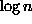diverges as n gets large,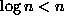for all integers. Hence,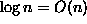. Furthermore, as the following theorem will show,raised to any integer power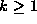is still O(n).

Theorem  For every integer,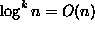.

extbfProof This result follows immediately from Theoremand the observation that for all integers,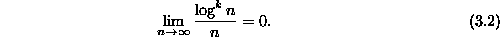This observation can be proved by induction as follows:

Base Case Consider the limit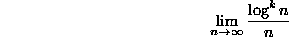for the case k=1. Using L'Hôpital's rulewe see that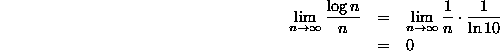Inductive Hypothesis Assume that Equationholds for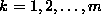. Consider the case k=m+1. Using L'Hôpital's rule  we see that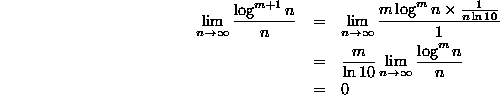Therefore, by induction on m, Equationholds for all integers.

For example, using this property of logarithms together with the rule for determining the asymptotic behavior of the product of two functions (Theorem), we can determine that since, then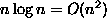.Copyright © 2001 by Bruno R. Preiss, P.Eng. All rights reserved.